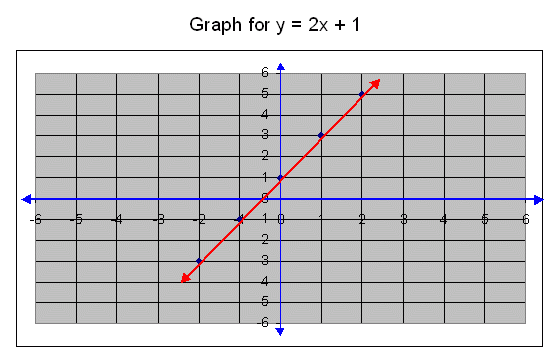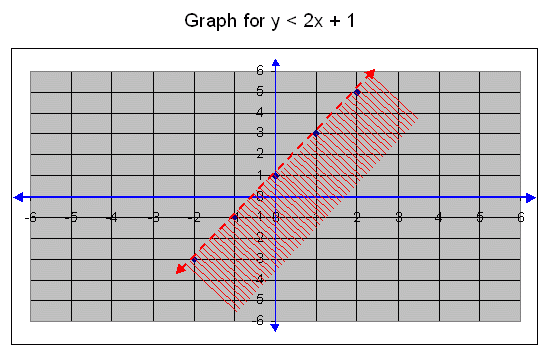Name: ___________________Date:___________________

kwizNET Subscribers, please login to turn off the Ads!
 Email us to get an instant 20% discount on highly effective K-12 Math & English kwizNET Programs!

### Middle/High School Algebra, Geometry, and Statistics (AGS)2.15 Graph for y < 2x+1

Draw the graph for y < 2x + 1.

First we draw a straight line for y = 2x + 1.
The ordered pairs for the graph y = 2x + 1.
x-2-1012
y = 2x + 1-3-1135
Ordered pairs(-2,-3)(-1,-1)(0,1)(1,3)(2,5)In the above graph the straight line separates the plane into two regions and every point on the straight line satisfies the equation y = 2x + 1.?
To find out the region for y < 2x+1, first we want to find out that the origin is lies in the region or not.
Substitute x = 0 and y = 0 in the inequation,
o < 2.0+1
0 < 1.
Therefore the origin lies in the half place.In the above graph the boundary line y = 2x + 1 does not lies in the region. That way we show that the boundary indicated in dotted line.

Directions: Draw the graphs for: y < 2x + 2, y < 2x + 3, y < 2x + 5, y < 2x + 12, and y < 2x + 6.
Also write at least five examples/graphs of your own.